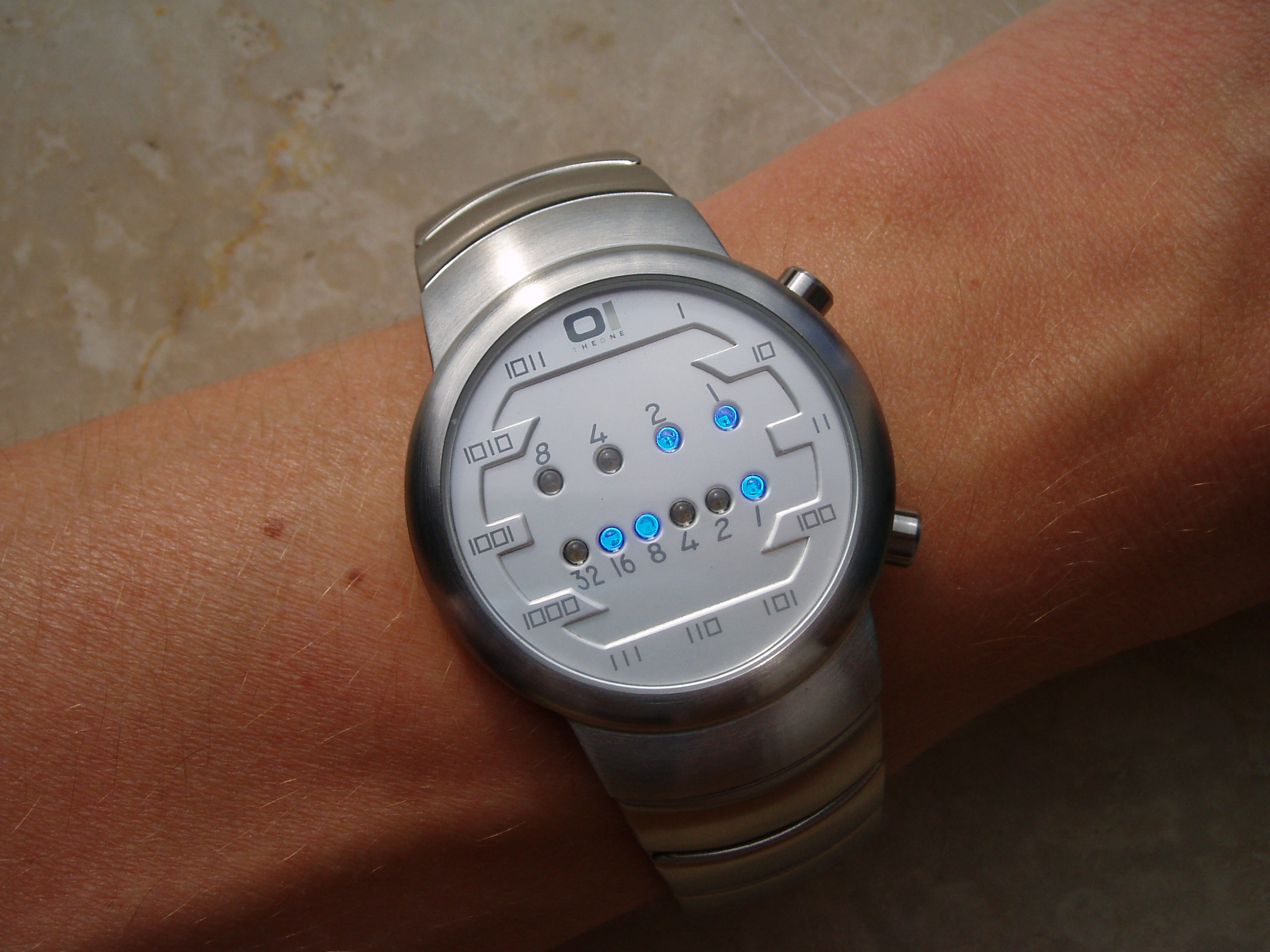@Yano 2017-12-10T02:01:23.000000Z 字数 1363 阅读 1557

# LeetCode 401 Binary Watch

LeetCode

# 题目描述

A binary watch has 4 LEDs on the top which represent the hours (0-11), and the 6 LEDs on the bottom represent the minutes (0-59).

Each LED represents a zero or one, with the least significant bit on the right.For example, the above binary watch reads "3:25".

Given a non-negative integer n which represents the number of LEDs that are currently on, return all possible times the watch could represent.

Example:

Input: n = 1
Return: ["1:00", "2:00", "4:00", "8:00", "0:01", "0:02", "0:04", "0:08", "0:16", "0:32"]


Note:

The order of output does not matter.
The hour must not contain a leading zero, for example "01:00" is not valid, it should be "1:00".
The minute must be consist of two digits and may contain a leading zero, for example "10:2" is not valid, it should be "10:02".


# 代码

public List<String> readBinaryWatch(int num) {    List<String> result = new ArrayList<>();    int[] hour = {8, 4, 2, 1};    int[] minute = {32, 16, 8, 4, 2, 1};    for(int i = 0; i <= num; i++) {        List<Integer> hours = gen(hour, i);        List<Integer> minutes = gen(minute, num - i);        for(int h : hours) {            if(h > 11) continue;            for(int m : minutes) {                if(m > 59) continue;                result.add(h + ":" + (m < 10 ? "0" : "") + m);            }        }    }    return result;}private List<Integer> gen(int[] nums, int count) {    List<Integer> res = new ArrayList<>();    robot(nums, count, 0, 0, res);    return res;}private void robot(int[] nums, int count, int pos, int out, List<Integer> res) {    if(count == 0) {        res.add(out);        return;    }    for(int i = pos; i < nums.length; i++) {        robot(nums, count - 1, i + 1, out + nums[i], res);    }}• 私有
• 公开
• 删除